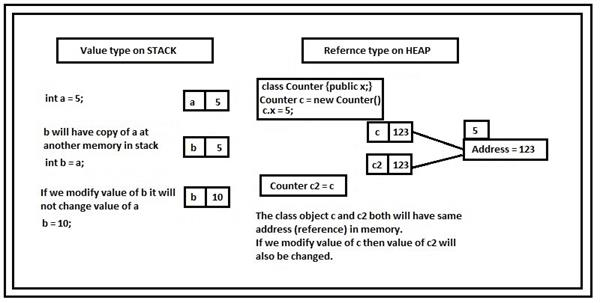Loading, please wait...# BOXING AND UNBOXING IN C#

May 14, 2020 C#, CSharp, Boxing and Unboxing, 2914 Views
We would learn the boxing and unboxing technique in C#. Before learning about boxing and unboxing in C# we need to learn about value type and reference type in C#.

### VALUE TYPES AND REFERENCE TYPES

• Value types are the primitive data types in C# which consist of the data at its own location in memory whereas reference types are the non-primitive data types in C# which consist of a pointer to another memory location holding the data.
• For value types, memory is allocated on the stack data structure. On the other hand, for reference types memory is allocated on the heap data structure.
• All the predefined data types under the libraries of our language which comes under the value type category like int (Int32), float, bool (Boolean) are implemented as structures whereas all the predefined data types under the reference type category like string and object are implemented as classes.

NOTE:- It should be noted that all the primitive data types are value type except string and object which are reference types.### BOXING AND UNBOXING

The terms  ‘boxing’ and ‘unboxing’ can be very easily understood if we correlate it with real times. For instance, boxing stuff in real-time would result in larger stuff because of the addition of packaging. Analogous to this, boxing in C# results in the transformation of a smaller data type (i.e. a subtype) to a larger data type (i.e. a supertype). Similarly, unboxing stuff results in smaller stuff because of the deduction of packaging. Likewise, unboxing in C# refers to the conversion of a larger data type(i.e. a supertype) into a smaller data type (i.e. a subtype).

In other words, we can say that boxing is a process that leads to the conversion from value type to reference type, and unboxing is a process that leads to the conversion from a reference type to a value type.

An important point to remember is that boxing can be implicit but unboxing is always necessarily explicit.

CODE FOR BOXING

Let us implement the following code to get a much better idea about boxing.

``````using System;

class Demo
{
static void Main()
{
int i = 12;
object obj = i ;
i = 5;
Console.Writeline(i);
Console.Writeline(obj);
}
}``````

EXPLANATION :

In the above code, we have an integer variable i which is of value type and is implicitly converted to the object variable obj which is of reference type. Here a smaller data type i.e. a subtype is converted to a larger data type i.e. a supertype hence, the above code is an example of boxing.

CODE FOR UNBOXING

``````using System;

class Demo1
{
static void Main()
{
object obj = 10;
int i = Convert.ToInt32(obj);
Console.Writeline(i);
}
}``````

EXPLANATION :

In the above code, we have an object variable obj which is of reference type, and since it cannot be implicitly converted to the integer value so it is done explicitly using Convert.ToInt32() .  Here a larger data type i.e. a super type is converted to a smaller data type i.e. a sub type hence, the above code is an example of unboxing.

CONCLUSION :

In this article, we learned about boxing and unboxing understanding the value types and reference types in C#.